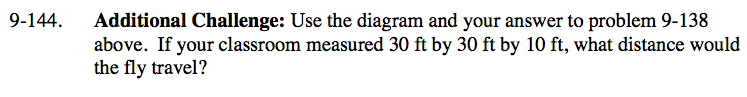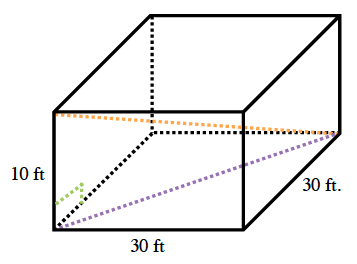Home > CC3 > Chapter 9 > Lesson 9.2.6 > Problem9-144

9-144.

Additional Challenge: Use the diagram and your answer to problem 9-138 above. If your classroom measured 30 ft by 30 ft by 10 ft, what distance would the fly travel? Homework Help ✎Find the distance the fly traveled by finding the hypotenuse of the triangle (in orange).
Notice that the longest leg of the right triangle (in purple) is the distance between two opposite corners on the floor.

$\sqrt{1900} \approx 43.59\text{ ft.}$